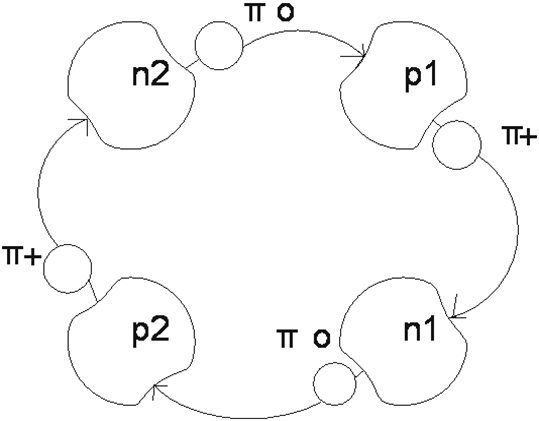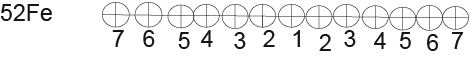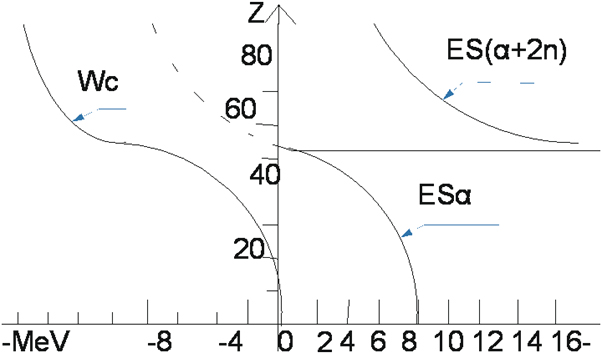## The structure of Atomic Nuclei

Buchakchiiskiy FF

University of St.Gagarin Severodonetsk, Ukraine

Buchakchiiskiy VS

Chernivtsi National University, 2, Kotsyubynskyi Str., Chernivtsi, 58012, Ukraine

Article
Article Info
Author Info
Figures & Data

## Abstract

All nuclei of elements consist of successive chain of nuclei of helium. Stability of nuclei of helium is provided by an exchange by mesons between nucleons. Division of uranium 235U takes place in a chain where value of energy of separation of nucleus of helium 4He close to the zero.

Successive chain of nuclei of helium or isotopes of nuclei of helium stability is provided by cooperation of nuclides.

## Introduction

It is known that the number of nucleons in a nuclei is multiple four plays a large role at determination of properties of nucleus Foremost at the nuclei of containing an even number protons and neutrons spin of nucleus equal to the zero. To this group of elements belong helium 4He,carbon 12C,oxygen 16O.Beecause the nucleus of helium 4 He is the simples ,then it serves as basis for the construction of all anther nuclei. .

#### Substantive Provisions

Basis (by a brick) for formation of nuclei of elements is a nucleus of helium 4He or isotope nucleus of helium. All other nuclei of elements consist of successive chain of nuclei of helium. Why did the nucleus of helium become basis all other nuclei?

Appearance of fourth sector (proton or neutron) in the chart of tritium (3H) or chart of isotope of helium (3He) creates the complete structure of nucleus of helium. Thus sharply the size of binding energy grows to 28.296 MeV and binding energy on nuclide to 7.04 MeV. And no another way thus to increase the value of binding energy exists. Therefore the nucleus of helium becomes basis for all elements.

The nucleus of helium consists of two protons and two neutrons. A proton is a stable particle and neutron is unstable. How to provide stability of nucleus of helium? Stability of nucleus is provided by cooperation of protons and neutrons by means of π-mesons. We will consider   one of variants of such cooperation. (Figure 1)Figure 1.

How do nucleus cooperate in a nucleus? They are constrained and cooperate all together. Protons and neutrons are difficult systems consisting of central part and cloud of the continuously emitted and taken in mesons. The proton P1 emits a π+meson and he is taken in by the neutron n1.The proton p2 emits the same π+meson, that is taken in by the neutron n2. And at same time neutrons emit π-0 mesons. That is taken in by protons .After an exchange of the mesons proton and neutron change by rotes.  A proton becomes a neutron and former neutron by a proton. This cooperation recurs in the next loop. A current flows in the ring of helium, because the charge of 1e moves, that creates the magnetic field. This magnetic field helps the orientation of nucleus of helium in relation to other nuclei.

The nuclei of elements consist of chain of nuclei of helium that is bound by inter se binding energy of equal energy of separation of nucleus of helium. This connection come a true by a transmission between nuclei of part of energy. This cooperation connection ends with in nuclei. Why does this chain coagulate in a ball? Maybe she aims to occupy a minimum volume in space.

Why do the nuclei of all elements consist of a sequential chain of helium nuclei? We start by analyzing the helium core. We divide the helium core into two equal parts. To find the energy of   separation of a part of the nucleus from the whole nucleus it is necessary to subtract from the binding energy of the whole nucleus the binding energy of its two parts.  BE-binding energy, ES-energy of separation.    ES (2H) (4He) =BE(4He)-BE(2H)-(BE2H)=28.296-2.225-2.225=23.846MeV.

Next, we will study the 52Fe core .To analyze the 52Fe core we will use the same principle as for the helium core. Although this method is applicable with restrictions for the 52Fe the following factors are not taken into sccount: 1) the shape of the nucleus 2) the influence of a positive charge. Divide the 52FE core into two equal parts.

1). ES (26Al) (52Fe)=BE(52Fe)-BE(26AL)-BE(26Al)=447.705-211.892-211.892=23.921MeV

We obtained a value almost equal to the value of the division of the helium nucleus into two parts We assume that the helium nucleus is located in the center of the 52Fe nucleus. (Figure 2)Figure 2.

2).Next, we divide the 52Fe core into unequal parts. One part is 26Na and the second part is 30P.

ES (22Na) (52Fe)=BE(52Fe)-BE(22Na)-BE(30P)=447.705-174.148-250.409=23.148

Again, a value comparable to the fission of the helium nucleus was obtained. In this case we have a division into two  parts 22Na and  30P.One fission point is located to the  left  of the center of the  nucleus  and the second to the  right of the center Thus , we already have three parts of the 52Fe nucleus consisting of  helium nuclei..  (Figure 3)Figure 3.

3). Now we divide the 52Fe core into two parts consisting of 18F and 34Cl.

ES (18F) (52Fe)=BE(52Fe)-BE(18F)-BE(34CL)=447.705-137372-285.568=24.765MeV (Figure 4)Figure 4.

Now already have five parts of  the 52Fe nucleus.

4). Let us do four more calculations similar to those done above.

ES (14N)(52Fe)=BE9(52Fe)-BE(14N)-BE(38K)=447.705-104.661-320.650=22.394MeV (Figure 5)Figure 5.

5). ES (10B) (52Fe)=BE(52Fe)-BE(10B)-BE(42Sc)=447.705-64.753-354.692=28.260MeV

6).ES (6Li) (52Fe)=BE(52Fe)-BE(6Li)-BE(46V)=447.705-31.995-390.365=25.345MeV

7).ES (2H) (52Fe)=BE(52Fe)-BE(2H)=BE(50Mn)=447.705-2.225-426.640=18.840MeV

Thus, we got all 13 helium nuclei that make up the 52Fe nucleus. (Figure 6)

BE (52Fe) =13х28.296+(-0.092)+7.367+7.162+4.725+9.322+9.985+6,947+6.641+7.050+5.125+7.696+7.937=447.705Me (Figure 6) (Table 1)Figure 6.

Table 1.  Formation of Nuclei of 235U and 238U

 92 184 U 220 221 222 223 224 225 226 227 228 229 230 231 232 233 234 235 238 90 180 229 232 5.710 88 176 223 226 4.538 86 172 217 220 8.501 84 168 211 214 6.253 82 164 205 208 10.998 80 160 199 202 12.650 54 78 156 193 196 10.636 48 49 50 51 52 53 76 152 187 190 10.591 187 190 74 148 35 36 37 38 39 40 41 42 43 44 45 46 47 181 184 72 144 176 178 9.180 70 140 171 172 5.770 68 136 166 167 6.500 66 132 161 162 9.454 35 36 37 38 64 128 156 157 9..359 62 124 150 151 152 5.036 60 120 144 146 147 5.006 58 116 140 141 142 8.606 56 112 136 137 16.763 54 108 131 16.792 52 104 125 16.550 50 100 119 20.699 119 48 96 18.200 113 46 92 20 21 22 23 24 25 26 27 28 29 30 31 32 107 ES(a+2n) = 18.07 44 88 101 ES (a+n) =9.666 42 84 96 2.761 40 80 92 2.198 38 76 88 7.916 36 72 84 ES(α+n) =17.904 34 68 79 22.220 32 64 73 21.013 30 50 67 ES(α+2n) =21.254 28 56 61 ES(α+n) =15.005 26 52 56 7.614 24 48 52 7.696 22 44 48 5.125 20  40 44 ES(α)=7.050 18 36 40 ES(a+2n) =26.033 16 32 34 ES(α+2n) =15.302 14 28 28 9.985 12 24 24 9.322 10 20 20 4.730 8 16 16 7.162 6 12 12 7.367 4 8 8 ES(α)=0.092 Z 2Z n 1 2 3 4 5 6 7 8 9 10 11 12 13 14 15 16 17 18 19

Why are the 235U uranium nucleus divided and the 238U nucleus not divided? Where is the fission point? The uranium nucleus consists of 46 helium nuclei or helium nuclei isotopes. They are interconnected  by  the binding energy which we traditionally call the separation energy  ES(a) or ES(a+n)or ES(a+2n).The magnitude of this energy depends on the following factors:1)the magnitude of Z  2)the type of  helium nucleus 3) the  magnitude of the Coulomb energy. (Figure 7) (Table 2 and 3 table)Figure 7.

Table 2.

 64 ES (a+2n) =13.080 156 157 ES (a+1n) =7.403MeV 62 ES (a+2n) =10.900 150 152 ES (a+1n) =8.003 60 ES (a) =   -1.012 144 147 ES (a+1n) =5.006 58 ES (a) =1.629b 140 142 ES (a+1n) =8.606 56 ES (a+n) =11.401 136 137 ES (a+2n) =16.388MeV 54 131

Table 3.

 64 ES (a+n) = 9.366 156 167 ES (a+1n) =7.403MeV 62 ES (a+n) =5.003 151 152 ES (a+1n) =8.003 60 ES (a+n) =6.880 146 147 ES (a+1n) =5.008 58 ES (a)    =0.144 141 142 ES (a+1n) =8.206 56 ES (a+2n) =16.388 137 ES (a+2n) =16.388MeV 54 131

Table1 shows the formation scheme of the 235U and 238U isotopes. It is known that the 235U isotope is divided into two parts and the 238U isotope is not divided. What is the difference?

Let us consider in more detail. Tabl.2 (part of Tabl1) shows the path to the sequential formation of the a uranium element from Z54 to Z64.Prior to A131 the formation of  the 235U and 238U isotopes proceeds along  the same  branch. Starting with A131 the isotope paths diverge Isotope 235U goes along the branches A131 A136 A140 A14 ,A15 ,A156 and further along the left branch to the end.

At the A136 A140 transition the binding energy  between helium nuclei becomes 1.629MeV and at theA140,A144 transition the binding energy  becomes negative ES(a)=-1.012MeV. This is the transition which is a weak point and causes the division of the 235U isotopes. The formation of 238U isotope proceeds along the path A131,A137,A142,A147 A152,A157,A162,A167 and  further along the right branch to A238. There are no net places in this branch.The second variant of the formation of these isotopes in Tabl.3.Prior to A137 the formation 0f 235U and 238U isotopes proceeds along the same branch. Beginning with A137 the isotope paths diverge. The 238U isotope follows the same path and the 235U isotope follows the A137 A141 A146 A151, A156 path and further along the previous branch to the end. There is a weak point in the transition between A137 and A141. The binding energy between  the helium nuclei here is   0.144MeV .The second  variant of the  formation of the 235U nucleus is more likely. This is the place where the chain can be broken into two parts by a thermal nutron.

## Conclusion

Dividing of uranium by two parts making 2/3 basic nucleus takes place the break of successive chain of nucleus where a value ES (a) approaches a zero.

## References

1. Buchakchiiskiy FF (2018) Model of Nuclear. J Phys Astron  Rev  6 : 1. [crossref]
2. Buchakchiiskiy FF (2019) About the model of nuclear. 4: 2.
3. Global National Data Center 2017.
4. Centre for Photonuclear Experiments 2017. [crossref]

Review Article

#### Publication history

Accepted date: June 16, 2020
Published date: June 19, 2020

©2020 Buchakchiiskiy FF. This is an open-access article distributed under the terms of the Creative Commons Attribution License, which permits unrestricted use, distribution, and reproduction in any medium, provided the original author and source are credited.

#### Citation

Buchakchiiskiy FF. (2020) The structure of Atomic Nuclei. OSP J Nuc Sci 2: JNS-2-112

#### Buchakchiiskiy FF

University of St.Gagarin Severodonetsk, Ukraine
ffbuchak@gmail.comFigure 1.Figure 2.Figure 3.Figure 4.Figure 5.Figure 6.Figure 7.

Table 1.  Formation of Nuclei of 235U and 238U

 92 184 U 220 221 222 223 224 225 226 227 228 229 230 231 232 233 234 235 238 90 180 229 232 5.710 88 176 223 226 4.538 86 172 217 220 8.501 84 168 211 214 6.253 82 164 205 208 10.998 80 160 199 202 12.650 54 78 156 193 196 10.636 48 49 50 51 52 53 76 152 187 190 10.591 187 190 74 148 35 36 37 38 39 40 41 42 43 44 45 46 47 181 184 72 144 176 178 9.180 70 140 171 172 5.770 68 136 166 167 6.500 66 132 161 162 9.454 35 36 37 38 64 128 156 157 9..359 62 124 150 151 152 5.036 60 120 144 146 147 5.006 58 116 140 141 142 8.606 56 112 136 137 16.763 54 108 131 16.792 52 104 125 16.550 50 100 119 20.699 119 48 96 18.200 113 46 92 20 21 22 23 24 25 26 27 28 29 30 31 32 107 ES(a+2n) = 18.07 44 88 101 ES (a+n) =9.666 42 84 96 2.761 40 80 92 2.198 38 76 88 7.916 36 72 84 ES(α+n) =17.904 34 68 79 22.220 32 64 73 21.013 30 50 67 ES(α+2n) =21.254 28 56 61 ES(α+n) =15.005 26 52 56 7.614 24 48 52 7.696 22 44 48 5.125 20  40 44 ES(α)=7.050 18 36 40 ES(a+2n) =26.033 16 32 34 ES(α+2n) =15.302 14 28 28 9.985 12 24 24 9.322 10 20 20 4.730 8 16 16 7.162 6 12 12 7.367 4 8 8 ES(α)=0.092 Z 2Z n 1 2 3 4 5 6 7 8 9 10 11 12 13 14 15 16 17 18 19

Table 2.

 64 ES (a+2n) =13.080 156 157 ES (a+1n) =7.403MeV 62 ES (a+2n) =10.900 150 152 ES (a+1n) =8.003 60 ES (a) =   -1.012 144 147 ES (a+1n) =5.006 58 ES (a) =1.629b 140 142 ES (a+1n) =8.606 56 ES (a+n) =11.401 136 137 ES (a+2n) =16.388MeV 54 131

Table 3.

 64 ES (a+n) = 9.366 156 167 ES (a+1n) =7.403MeV 62 ES (a+n) =5.003 151 152 ES (a+1n) =8.003 60 ES (a+n) =6.880 146 147 ES (a+1n) =5.008 58 ES (a)    =0.144 141 142 ES (a+1n) =8.206 56 ES (a+2n) =16.388 137 ES (a+2n) =16.388MeV 54 131